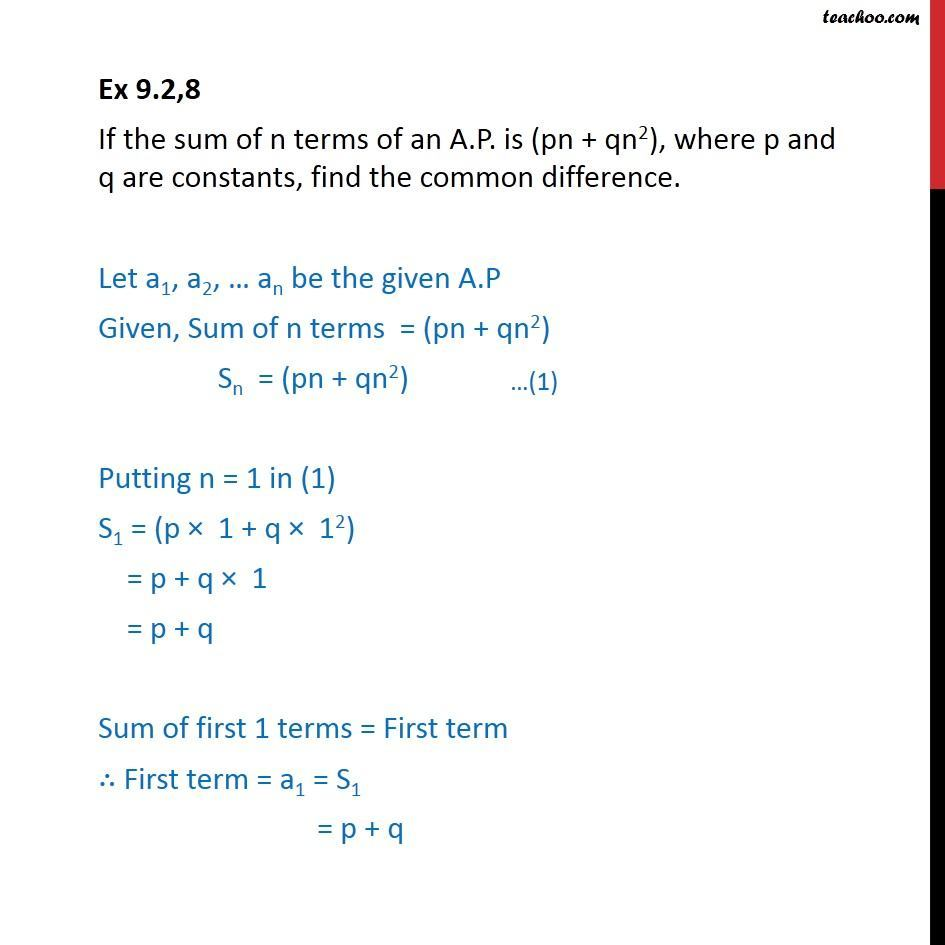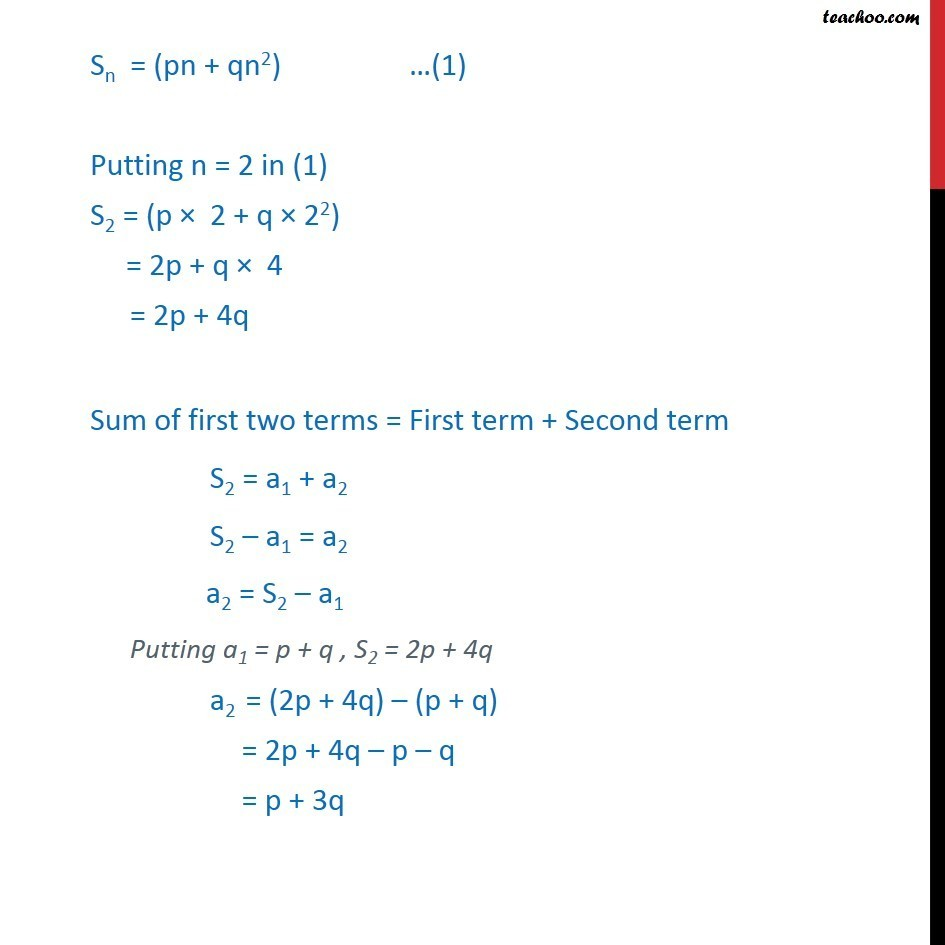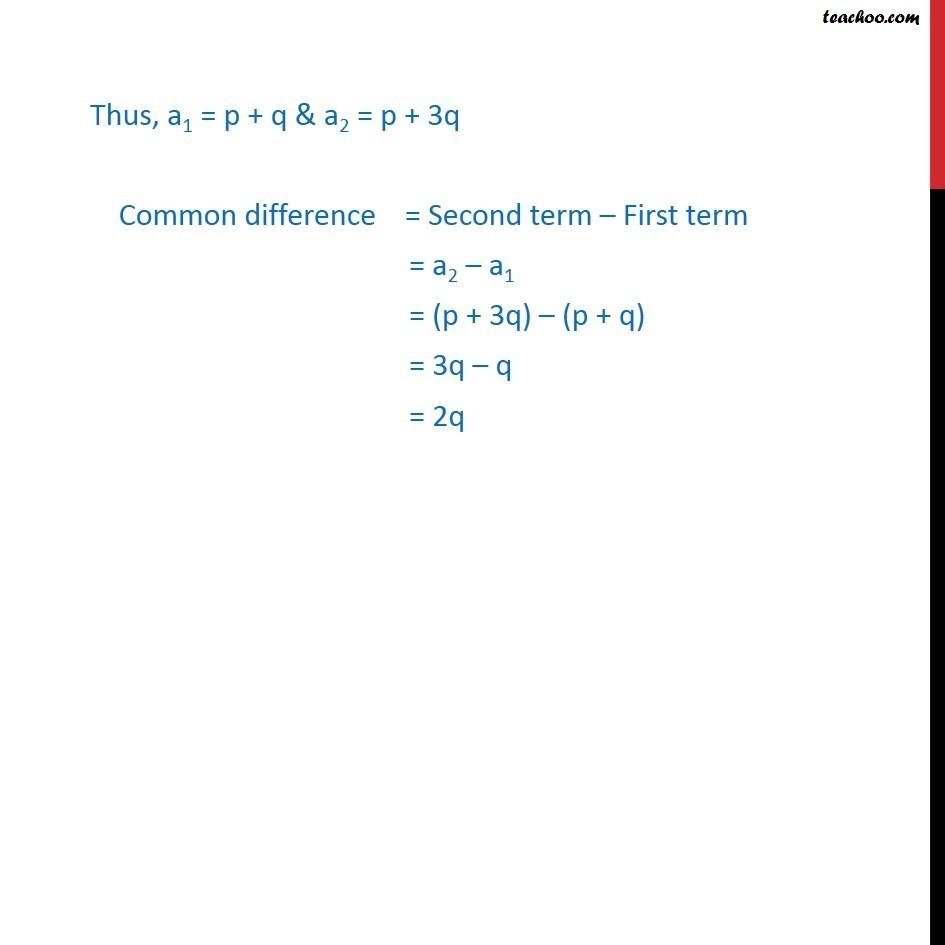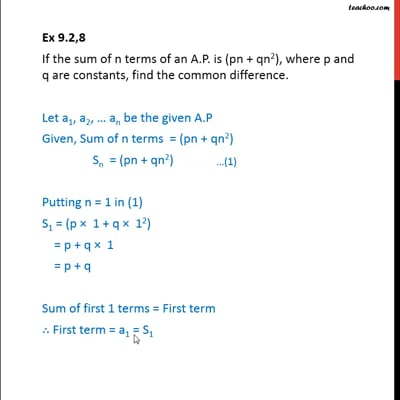Ex 9.2

Chapter 9 Class 11 Sequences and Series (Term 1)
Serial order wiseThis video is only available for Teachoo black users

### Transcript

Ex 9.2,8 If the sum of n terms of an A.P. is (pn + qn2), where p and q are constants, find the common difference. Let a1, a2, an be the given A.P Given, Sum of n terms = (pn + qn2) Sn = (pn + qn2) Putting n = 1 in (1) S1 = (p 1 + q 12) = p + q 1 = p + q Sum of first 1 terms = First term First term = a1 = S1 = p + q Sn = (pn + qn2) (1) Putting n = 2 in (1) S2 = (p 2 + q 22) = 2p + q 4 = 2p + 4q Sum of first two terms = First term + Second term S2 = a1 + a2 S2 a1 = a2 a2 = S2 a1 Putting a1 = p + q , S2 = 2p + 4q a2 = (2p + 4q) (p + q) = 2p + 4q p q = p + 3q Thus, a1 = p + q & a2 = p + 3q Common difference = Second term First term = a2 a1 = (p + 3q) (p + q) = 3q q = 2q